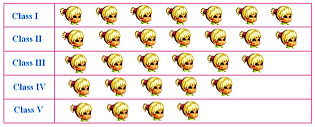Mathematics
Easy

Question

# How many girls are there in class 4?## 5468Hint:

## The correct answer is: 5

###Here we have to find the number of girls in class 4,Firstly, In fig, we given by each classes and also the number of  girls,On class 4 , see on table then,Count the total number of girls,Therefore, the total number of girls in class 4 is 5.The correct answer is 5 (option (a)).

In this question , We have given by table we just find out the given classes and count the number of girls in the row.

### Related Questions to study#### With Turito Foundation.#### Get an Expert Advice From Turito.